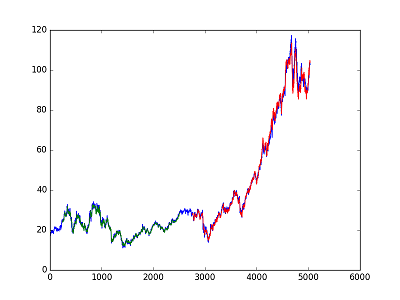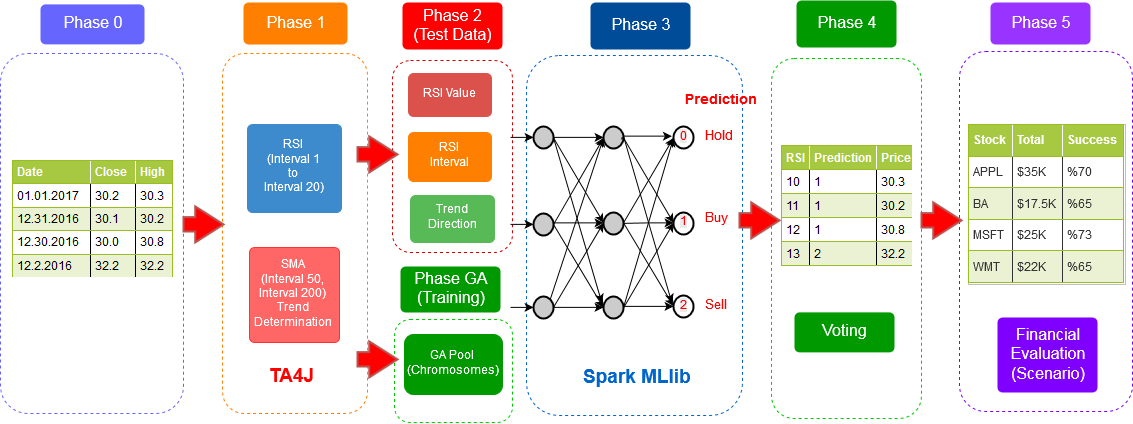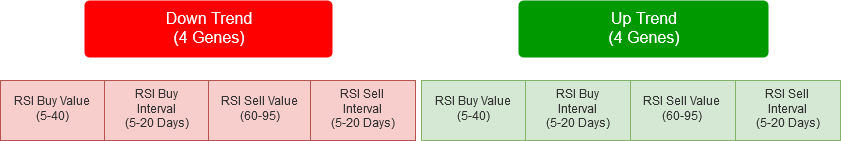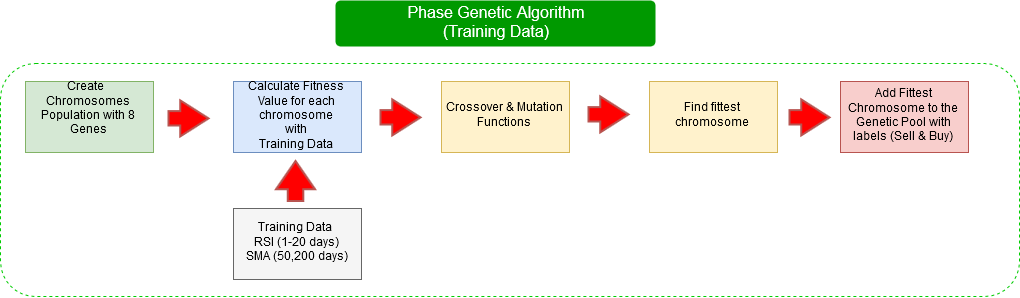## Prediction of Stock Prices Using LSTM network

Stock and ETFs prices are predicted using LSTM network (Keras-Tensorflow).

• Closed value (column) is used in the network.
• Values are normalized in range (0,1).
• Datasets are splitted into train and test sets, 50% test data, 50% training data.
• Keras-Tensorflow is used for implementation.
• LSTM network consists of 25 hidden neurons, and 1 output layer (1 dense layer).
• LSTM network features input: 1 layer, output: 1 layer , hidden: 25 neurons, optimizer:adam, dropout:0.1, timestep:240, batchsize:240, epochs:1000 (features can be further optimized).
• Root mean squared errors are calculated.
• Output files: lstm_results (consists of prediction and actual values), plot file (actual and prediction values).What is LSTM? (General Information) https://en.wikipedia.org/wiki/Long_short-term_memory

Keras: https://keras.io/

Tensorflow: https://www.tensorflow.org/

## A Deep Neural-Network based Stock Trading System based on Evolutionary Optimized Technical Analysis Parameters

In this study, we propose a stock trading system based on optimized technical analysis parameters for creating buy-sell points using genetic algorithms. The model is developed utilizing Apache Spark big data platform. The optimized parameters are then passed to a deep MLP neural network for buy-sell-hold predictions. Dow 30 stocks are chosen for model validation. Each Dow stock is trained separately using daily close prices between 1996-2016 and tested between 2007-2016. The results indicate that optimizing the technical indicator parameters not only enhances the stock trading performance but also provides a model that might be used as an alternative to Buy and Hold and other standard technical analysis models. The phase of proposed method is illustrated in below.Utilizing optimized technical analysis feature parameter values as input features for neural network stock trading system is the basis for our proposed model. We used genetic algorithms to optimize RSI parameters for uptrend and downtrend market conditions. Then, we used those optimized feature values as buy-sell trigger points for our deep neural network data set. We used Dow 30 stocks to validate our model. The results indicate that such a trading system produces comparable or better results when compared with Buy & Hold and other trading systems for a wide range of stocks even for relatively longer periods. The structure of the chromosomes and genes in the chromosomes are shown below.

• RSI Buy values are created randomly between 5 and 40.
• RSI Buy intervals are created randomly between 5 and 20 days.
• RSI Sell values are created randomly between 60 and 95.
• RSI Sell intervals are created randomly between 5 and 20 days.
• The same procedure is followed to create 4 genes for uptrend.Genetic algorithm phase is illustrated as follows:Cite as:

Bibtex:

``````@article{sezer2017deep,
title={A Deep Neural-Network Based Stock Trading System Based on Evolutionary Optimized Technical Analysis Parameters},
author={Sezer, Omer Berat and Ozbayoglu, Murat and Dogdu, Erdogan},
journal={Procedia Computer Science},
volume={114},
pages={473--480},
year={2017},
publisher={Elsevier}
}
``````

MLA:

Sezer, Omer Berat, Murat Ozbayoglu, and Erdogan Dogdu. “A Deep Neural-Network Based Stock Trading System Based on Evolutionary Optimized Technical Analysis Parameters.” Procedia Computer Science 114 (2017): 473-480

What is Multi Layer Perceptron (MLP)? (General Information): https://en.wikipedia.org/wiki/Multilayer_perceptron

What is Genetic Algorithm?: https://en.wikipedia.org/wiki/Genetic_algorithm

What is Relative Strength Index?: https://en.wikipedia.org/wiki/Relative_strength_index

Apache Spark MLlib: https://spark.apache.org/mllib/